# ASSET Practice Test

If you’ve been thinking of going to college, you may have run across references to something called the ASSET test, or simply ASSET. This is a test that you may have to take before starting school. Of course, if you’re like most people, you haven’t heard of ASSET. Almost everyone who considers going to college has heard of the ACT exam or the SAT test, but not this particular test. That’s because the number of schools that use this test is tiny compared to the number that use either the SAT or ACT.

ASSET is actually administered by ACT, Inc., which is the company that administers the ACT test. (ACT doesn’t officially stand for anything; years ago the initials stood for American College Testing, but like Kentucky Fried Chicken officially changing its name to KFC, ACT became the legal name of the company a few years ago.)

However, it serves a different purpose than the ACT test. The ACT, which is used by thousands of colleges and universities, is more academically challenging than the ASSET exam. Most schools that use the ACT are four year colleges and universities, while the ASSET exam is used primarily by technical schools and two year community colleges (AKA “junior colleges”).

Of course, not all two year colleges and technical schools require incoming students to take the ASSET, but many do. If you’re attending a school that uses this test, the school will use your score to help determine what level of academic challenge you’re prepared for in several areas. College administrators want to make sure that students get the education they’re paying for, which means it’s important that there’s not a mismatch between their skill levels and the work they’re being asked to do.

If a student is being given assignments he’s not ready for, he will likely fall behind in his studies and not graduate on time, and odds are high that he’ll simply drop out altogether. Then he’ll be stuck paying off college loans for an education he didn’t get. On the other hand, if a student is being given assignments that are too easy, which don’t challenge him at all, he will become bored.

He’ll realize his time is being wasted, and he will also be at high risk for dropping out, after deciding that college is a “joke” and a “ripoff” because he wasn’t learning anything new. That’s why it’s so important to place students in classes closely suited to their skills and knowledge levels, and the ASSET exam helps colleges do just that.

## Practice Questions

### Writing Skills

Each underlined word or phrase in the following passage either does or doesn’t contain an error or style, grammar, or usage. If you believe it’s correct as shown, select a for NO CHANGE; otherwise, select the answer that would be the best choice to replace the word or phrase.

Once upon a time, (1) there was a village in the jungle, a man appeared and announced to the villagers that he would buy monkeys for \$10 each. (2) Seeing as how there were many monkeys around, the villagers went out to the (3) forest, and started catching them. The man bought thousands at \$10, (4) and, as the supply started to diminish, the villagers let (5) there efforts lag. The man (6) later announced that he would buy monkeys at \$20 each. This renewed the vigor of the (7) villagers and got them catching monkeys again.

##### 1. there was
1. NO CHANGE
2. there was once
3. in
4. there is
##### 2. Seeing as how
1. NO CHANGE
2. Since
3. Seeing that
4. Noticing that
1. NO CHANGE
2. forest and
3. woods, and
4. skillfully
1. NO CHANGE
2. since
3. when
4. but
##### 5. there
1. NO CHANGE
2. OMIT this word
3. they’re
4. their
##### 6. later
1. NO CHANGE
2. OMIT this word
3. soon
4. kindly
##### 7. villagers and
1. NO CHANGE
2. villagers though
3. townspeople, and
4. villagers, and

Daylight Saving Time (DST) is the practice of changing clocks so that afternoons have more daylight and mornings have less. Clocks are adjusted forward one hour in the spring and one hour backward in the fall. The main purpose of the change is to make better use of daylight.

DST began with the goal of conservation. Benjamin Franklin suggested it as a method of saving on candles. It was used during both World Wars to save energy for military needs. Although DST’s potential to save energy was a primary reason behind its implementation, research into its effects on energy conservation are contradictory and unclear.

Beneficiaries of DST include all activities that can benefit from more sunlight after working hours, such as shopping and sports. A 1984 issue of Fortune magazine estimated that a seven-week extension of DST would yield an additional \$30 million for 7-Eleven stores. Public safety may be increased by the use of DST: some research suggests that traffic fatalities may be reduced when there is additional afternoon sunlight.

On the other hand, DST complicates timekeeping and some computer systems. Tools with built-in time-keeping functions such as medical devices can be affected negatively. Agricultural and evening entertainment interests have historically opposed DST.

DST can affect health, both positively and negatively. It provides more afternoon sunlight in which to get exercise. It also impacts sunlight exposure; this is good for getting vitamin D, but bad in that it can increase skin cancer risk. DST may also disrupt sleep.

Today, daylight saving time has been adopted by more than one billion people in about 70 countries. DST is generally not observed in countries near the equator because sunrise times do not vary much there. Asia and Africa do not generally observe it. Some countries, such as Brazil, observe it only in some regions.

DST can lead to peculiar situations. One of these occurred in November, 2007 when a woman in North Carolina gave birth to one twin at 1:32 a.m. and, 34 minutes later, to the second twin. Because of DST and the time change at 2:00 a.m., the second twin was officially born at 1:06, 26 minutes earlier than her brother.

##### 7. According to the passage, what is the main purpose of DST?
1. To increase public safety
3. To make better use of daylight
4. To promote good health
##### 8. Which of the following is not mentioned in the passage as a negative effect of DST?
1. Energy conservation
2. Complications with time keeping
3. Complications with computer systems
4. Increased skin cancer risk
##### 9. The article states that DST involves:
1. Adjusting clocks forward one hour in the spring and the fall
2. Adjusting clocks backward one hour in the spring and the fall
3. Adjusting clocks forward in the fall and backward in the spring
4. Adjusting clocks forward in the spring and backward in the fall
##### 10. Which interests have historically opposed DST, according to the passage?
2. evening entertainment and agriculture
3. 7-Eleven and health
4. medical devices and computing
##### 11. According to the article, increased sunlight exposure:
1. is always good for health
2. is always bad for health
3. has no effect on health
4. can be both good and bad for health

### Numerical Skills

1. 10
2. 50
3. 22
4. 18
1. 68
2. 85
3. 88
4. 84.8
1. \$850,000
2. \$4,000,000
3. \$1,150,000
4. \$650,000
##### 15. The sum of two negative numbers:
1. is always negative
2. is always positive
3. sometimes is positive and sometimes is negative
4. is always zero
1. 0
2. 1
3. 1/5
4. 5

### Elementary Algebra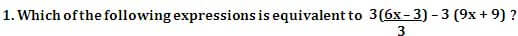1. -3(7x + 10)
2. -3x +6
3.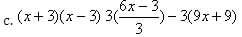4. 3x2 – 9
1. -1
2. +1
3. +4
4. -2
1. 9x -6
2. 9x -8
3. 27x -8
4. 27x -4
##### 4. Which of the following equations satisfies the five sets of numbers shown in the table?
 y -4 31 4 68 12 x -2 3 0 4 2
1. y = 2x2 + 7
2. y = x3 + 4
3. y = 2x
4. y = 3x + 1
1. 2
2. 3
3. 6
4. 12

### College Algebra

1. (5, 3)
2. (8, 13)
3. (-4, 13)
4. (-8, -13)
1. 4x2 + 11
2. 4x4 + 11
3. x2 + 11
4. 4 x2 + 14
##### 3. If x and y are positive integers, which of the following expressions is equivalent to (xy)7y – (xy)y ?
1. (xy) 6y
2. (xy) 7y-1
3. (xy)y [(xy)71:44 PM 9/11/2012 -1]
4. (xy)y[(xy)6y -1]
##### 4. Which equation is represented by the graph shown below?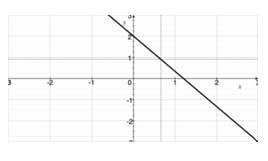1. y = 5 / 3 x + 2
2. y = – 5/3 x – 2
3. y = – 5/3 x + 2
4. y = 5/3 x -2
##### 5. The graph below, not drawn to scale, shows a straight line passing through the origin. Point P1 has the (x,y) coordinates (-5,-3). What is the x-coordinate of point P2 if its y-coordinate is 3?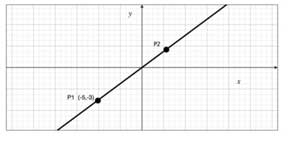1. 0.8
2. 1
3. 5
4. 3

### Geometry

1. πr 2
2. πr 2 / 2π
3. 2πr / r 2
4. r / 2
1. 10 units
2. 11 units
3. 12 units
4. 13 units
1. 10 units
2. 18 units
3. 24 units
4. 14 units
1. r2
2. 2r2
3. πr2
4. 2πr
##### 5. If the area of a right triangle is 20 ft2, which of the following represent the possible measurements for the base and height of the triangle?
1. B = 4 ft; H = 5 ft
2. B = 10 ft; H = 2 ft
3. B = 8 ft; H = 5 ft
4. B = 15 ft; H = 3 ft

### Writing Skills

1. C: Answer C as this phrase tells the reader where the man appeared. Answer A creates a run-on sentence, answer B is redundant, and choice D creates a disagreement of verb tense.

2. B: The original and answer C are slang usage, and D, suggesting that the villagers had only then noticed the monkeys, is inappropriate.

3. B: As the clause following the conjunction and is dependent, the comma is not employed.

4. D: The action described in the portion of the sentence following the conjunction is contrary to expectation, since the villagers hunted less despite the generous payments, and but reflects that contradiction better than any of the other choices.

5. D: The correct spelling for the possessive pronoun.

6. C: Answer C implies that the action that follows is a consequence of the one that precedes, i.e., the man raised his price because the villagers were losing interest.

7. A: No comma is used to set off a dependent clause.

7. C: The first paragraph states that the main purpose of DST it to make better use of daylight.

8. A: Energy conservation is discussed as a possible benefit of DST, not a negative effect of it.

9. D: The first paragraph states that DST involves setting clocks forward one hour in the spring and one hour backward in the fall.

10. B: The last sentence in paragraph four notes that agricultural and evening entertainment interests have historically been opposed to DST.

11. D: The passage gives examples of both good and bad effects extra daylight can have on health.

### Numerical Skills

1. C: 16.5 x 4/3 = 22.

6. B: The lowest score, 68, is eliminated. The average of the remaining four grades is (75 +88 + 86 + 90) divided by 4, which comes to 84.75. Rounding up to the nearest integer gives a final grade of 85. Since this value is unique, all the other answers are incorrect.

7. A: The band’s share, 25% of \$20,000,000, is \$5,000,000. After the agent’s share is subtracted, the band gets (1-0.15) x \$5,000,000 = 0.85 x \$5,000,000 = \$4,250,000 and each band member gets one fifth of that, or \$850,000.

15. A: Negative numbers represent segments extending to the left of zero on the number line. Adding a negative number to another negative number extends the segment even further to the left, or into “negative territory”. To add two negative numbers, add the magnitudes and retain the negative sign. For example (-3) + (-5) = -8.

17. B: The reciprocal of 5 is 1/5. When numbers are multiplied by their reciprocals, the result is always 1. Thus, 5 x (1/5) = 1.

### Elementary Algebra

1. A: From the starting expression, compute: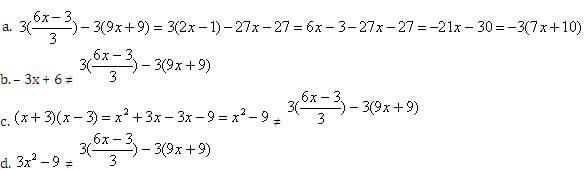2. B: Compute as follows: (3 – 2 x 2)2 = (3 – 4)2 = (-1)2 = 1.

3. C: Evaluate as follows:
(3x-2)3 = 33 x ( x -2)3 = 27 x (1 / x2)3 = 27x 1 / x8 = 27x -8

4. B: The easiest pair to test is the third: y = 4 and x = 0. Substitute these values in each of the given equations and evaluate. Choice B gives 4 = 0 + 4, which is a true statement. None of the other answer choices is correct this number set.

5. D: Solving for y in the second equation gives y = 4x-5. If we plug this into the first equation we get 2x + 3(4x-5) = 13. Solving for this equation gives us 14x = 28, or x = 2. Then, plug the value of x into either equation to solve for y. y = 3. Therefore, 3x + 2y = 12.

### College Algebra

1. D: At the point of intersection, the y-coordinates are equal on both lines so that 2x + 3 = x – 5. Solving for x, we have x = -8. Then, evaluating y with either equation yields
y = 2(-8) + 3 = -16 + 3 = -13 or y = -8 – 5 = -13

2. A: Evaluate as follows: 2f(x) – 3 = 2 (2x2 + 7) – 3 = 4x2 + 14 – 3 = 4x2 + 11.

3. D: Remember that when you multiply like bases, you add the exponents, and when you divide like bases, you subtract the exponents. (xy)7y – (xy)y = (xy)y [(xy)7y-y – 1] = (xy)y [(xy) 6y – 1]

4. C: The line in the graph has a negative slope and a positive y-axis intercept, so the factor multiplying the variable x, or the slope, must be negative, and the constant, or y-intercept, must be positive.

5. C: Since the line is straight, the slope is the same throughout. Thus, if 5 y-units are traversed in going from x = -3 to x = 0 (where y increases from -5 to 0, to reach the origin), then 5 y-units will be traversed in going from x = 0 to x = 3 .

### Geometry

1. D: The area of the circle is ?r2 while the circumference is 2?r. Taking the ratio of these two expressions and reducing gives: Ratio = πr2 / 2πr = r/2

2. D: The sides of a triangle must all be greater than zero. The sum of the lengths of the two shorter sides must be greater than the length of the third side. Since we are looking for the minimum value of the perimeter, assume the longer of the two given sides, which is 6, is the longest side of the triangle. Then the third side must be greater than 6 – 4 = 2. Since we are told the sides are all integral numbers, the last side must be 3 units in length. Thus, the minimum length for the perimeter is 4+6+3 = 13 units.

3. C: The hypotenuse must be the longest side of a right triangle, so it must be the lengths of the other two sides that are given as 6 and 8 units. Calculate the length of the hypotenuse, H, from the Pythagorean Theorem: H2 = S12 + S22 = 62 + 82 = 36 + 64 = 100 , which yields H = 10 and the perimeter equals 10+6+8 = 24.

4. A: The area of a triangle equals half the product of base times height. Since the base passes through the center, we have base = 2 r and height = r, so that the area A is

5. C: The formula for the area of a triangle is 1/2bh, or one-half the base times the height. This means that the product of the two sides will actually be twice the number that is provided as the area, i.e., 20 x 2 or 40. Although the actual base and height aren’t provided, only one answer choice offers two numbers that equal a product of 40 – 8 and 5.